# WBJEE 2019 Chemistry Paper with Solutions

Students will be able to view and download the WBJEE 2019 Chemistry question paper from this page. We are also providing step by step solutions for each of the question asked in the paper and these solutions have been prepared by specialized experts. The detailed solutions will help students to understand the correct answer and increase their speed of solving problems thereby boosting their confidence. This will further help them to be stress-free during the exam. Students can easily download the WBJEE 2019 question paper PDF for free and use it to practice offline.

### WBJEE 2019 - Chemistry

Question 1: The H–N–H angle in ammonia is 107.6°, while the H–P–H angle in phosphine is 93.5°. Relative to phosphine, the p-character of the lone pair on ammonia is expected to be:

1. a. Less
2. b. More
3. c. Same
4. d. Cannot be predicted

Solution:

As % s-character increases, bond angle increases

As % p-character increases, bond angle decreases.

Therefore, p-character order will be: PH3 > NH3

Therefore, the Bond angle order: PH3 < NH3

Question 2: The reactive species in chlorine bleach is

1. a. Cl2O
2. b. OCl
3. c. ClO2
4. d. HCl

Solution:

OCl is used as a bleaching agent. Its components will be: OCl →[O] + Cl

Question 3: The conductivity measurement of a coordination compound of Cobalt (III) shows that it dissociates into 3 ions in solution. The compound is

1. a. Hexaamminecobalt(III) chloride
2. b. Pentaamminesulphatocobalt(III) chloride
3. c. Pentaamminechloridocobalt(III) sulphate
4. d. Pentaamminechloridocobalt(III) chloride

Solution:

The compound is

Pentaaminechloridecobalt (III) chloride

$$[CO(NH_{3})_{5}Cl]\rightleftharpoons [CO(NH_{3})_{5}Cl^{-}]^{+2}+2Cl^{-}$$

Gives 3 ions in aqueous solution.

Question 4: In the Bayer's process, the leaching of alumina is done by using

1. a. Na2CO3
2. b. NaOH
3. c. SiO2
4. d. CaO

Solution:

The separation of the alumina from impurities is usually accomplished by Bayer’s process.

The reactions involved in this process are: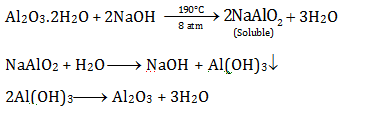Question 5: Which atomic species cannot be used as a nuclear fuel?

1. a.
$${ }^{233} _{92}{U}$$
2. b.
$${ }^{235} _{92}{U}$$
3. c.
$${ }^{239} _{94}{U}$$
4. d.
$${ }^{238} _{92}{U}$$

Solution:

Since

$${ }^{238} _{92}{U}$$
isotope of uranium does not participate in nuclear chain reaction, it cannot be used as nuclear fuel.

Question 6: The molecule/molecules that has/have delocalised lone pair(s) of electrons is/are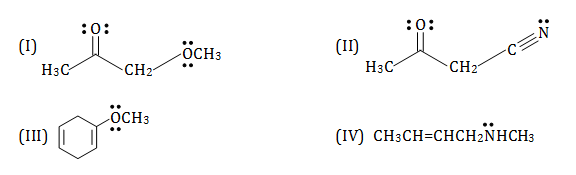1. a. I, II and III
2. b. I, II and IV
3. c. I and III
4. d. only III

Solution:

In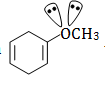there is π bond and lone pair conjugation so, the lone pair of oxygen get delocalized on π bond located on next carbon.

Question 7: The conformations of n-butane, commonly known as eclipsed, gauche and anti-conformations can be inter converted by

1. a. rotation around C-H bond of a methyl group
2. b. rotation around C-H bond of a methylene group
3. c. rotation around C1-C2 linkage
4. d. rotation around C2-C3 linkage

Solution: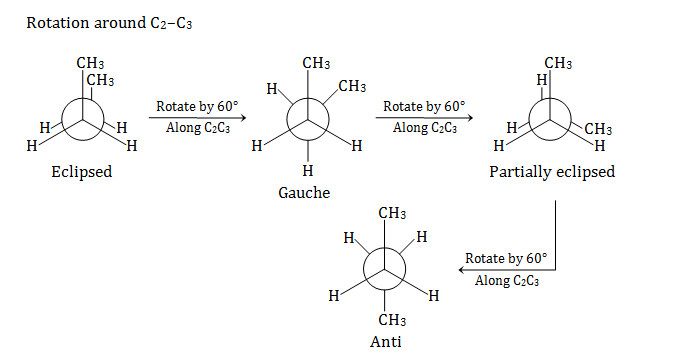Question 8: The correct order of the addition reaction rates of halogen acids with ethylene is

1. a. hydrogen chloride > hydrogen bromide > hydrogen iodide
2. b. hydrogen iodide > hydrogen bromide > hydrogen chloride
3. c. hydrogen bromide > hydrogen chloride > hydrogen iodide
4. d. hydrogen iodide > hydrogen chloride > hydrogen bromide

Solution:

Reactivity order is

HI > HBr > HCl

Therefore, Leaving group order is I> Br> Cl

The compound that has more stable leaving group will be more reactive with ethylene.

Question 9: One of the products of the following reactions P.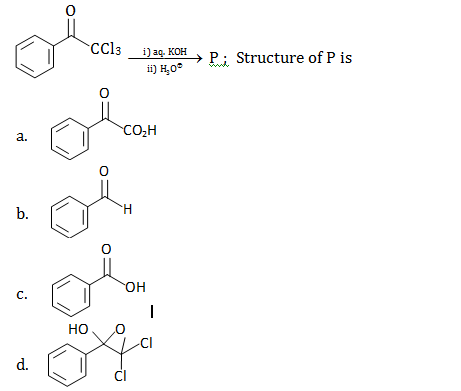Solution: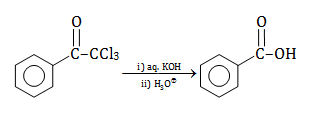Question 10: For the reaction below, the product is Q.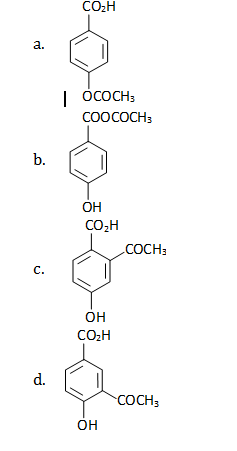Solution: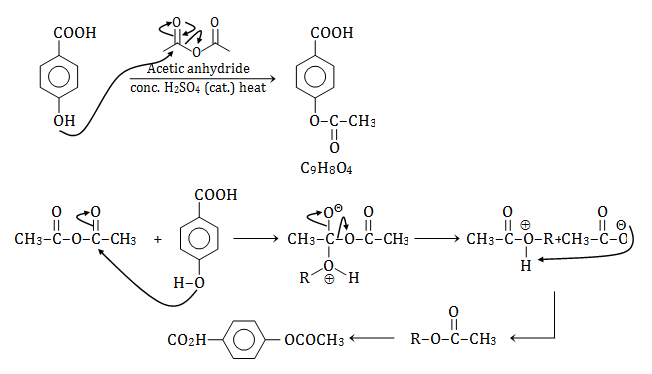Question 11: Cyclopentanol on reaction with NaH followed by CS2 and CH3I produces a/an

1. a. ketone
2. b. alkene
3. c. ether
4. d. xanthate

Solution: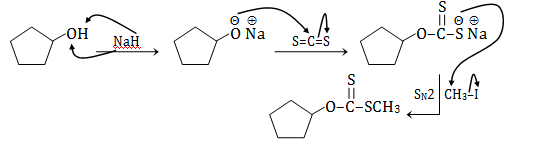Question 12: The compound, which evolves carbon dioxide on treatment with aqueous solution of sodium bicarbonate 25°C, is

1. a. C6H5OH
2. b. CH3COCl
3. c. CH3CONH2
4. d. CH3COOC2H5

Solution:

CH3COCl hydrolyses to form CH3COOH even at 25°C, which subsequently reacts with NaHCO3 present in the same medium to form CO2.

Question 13: The indicated atom is not a nucleophilic site in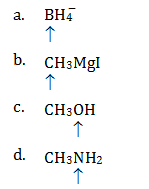Solution:

There is no lone pair on ‘B’ atom of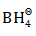, hence it is not nucleophilic site.

Question 14: The charge carried by 1 millimole of Mn+ ions is 193 coulombs. The value of n is

1. a. 1
2. b. 2
3. c. 3
4. d. 4

Solution:

Given: Charge on 1 millimole of Mn2+ ions = 193 C

So, 193 = (nx96500)/1000

Rearranging, n = (193 x 1000)/96500

So,

n = 2

Question 15: Which of the following mixtures will have the lowest pH at 298 K?

1. a. 10 ml 0.05NCH3COOH + 5 ml 0.1 NNH4OH
2. b. 5 ml 0.2NH4Cl + 5 ml 0.2 N NH4OH
3. c. 5 ml 0.1N CH3COOH + 10 ml 0.05 N CH3COONa
4. d. 5 ml 0.1N CH3COOH + 5 ml 0.1 N NaOH

Solution: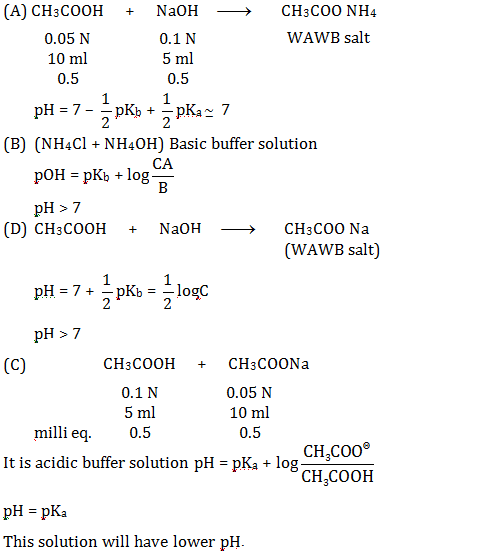Question 16: Consider the following two first-order reactions occurring at 298 K with same initial concentration of A:

(1) A →B: rate constant, k = 0.693 min–1

(2) A →C: half – life, t1/2 = 0.693 min–1

Choose the correct option:

1. a. Reaction (1) is faster than Reaction (2).
2. b. Reaction (1) is slower than Reaction (2).
3. c. Both the reaction proceeds at the same rate.
4. d. Since two different products are formed, rates cannot be compared.

Solution:

For 1st order reaction

Rate constant K = (0.693/t1/2) and Rate = K(A)’

For (I) reaction K = 0.6930 minute–1

For (II) reaction K =(0.693/t1/2) = (0.693/0.693) = 1 min–1

Since given t½ = 0.693

So, KI< KII

Then, Rate I < Rate II

Question 17: For the equilibrium

$$H_{2}O(l)\rightleftharpoons H_{2}O(g)$$
, which of the following is correct?

1. a. ΔG = 0, ΔH < 0, ΔS < 0
2. b. ΔG < 0, ΔH > 0, ΔS > 0
3. c. ΔG > 0, ΔH = 0, ΔS > 0
4. d. ΔG = 0, ΔH > 0, ΔS > 0

Solution:

For equilibrium

$$H_{2}O(l)\rightleftharpoons H_{2}O(g)$$

ΔG = 0 Since equilibrium

ΔH > 0 (+ve), for T =ΔH/ΔS the process will be spontaneous above the temperature of T

ΔS > 0 (+ve)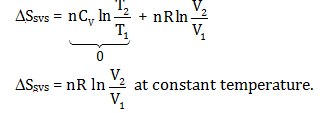Question 18: For a Vander Waal’s gas, the term (ab/v2) represents some

1. a. Pressure
2. b. Energy
3. c. Critical density
4. d. Molar mass

Solution:

Vander Waal’s equation is given as:

$$\left[{P}\left(1+\frac{{an}^{2}}{{V}^{2}}\right)\right]({V}-{nh})={nRT}$$

(ab/v2) represent energy by dimensional analysis.

Unit of a (Vander Waal’s constant) = atm.l2/mole2

Unit of b (Vander Waal’s constant) = l/mole

$$\frac{a b}{v^{2}}=\frac{\frac{a \operatorname{tm} \ell^{2}}{m o l e^{2}} \times \frac{\ell}{m o l e}}{\ell^{2} / m o l e^{2}}=\frac{a t m \ell}{m o l e}$$

Question 19: In the equilibrium

$$H_{2}+I_{2}\rightleftharpoons 2HI$$
, if at a given temperature the concentrations of the reactants are increased, the value of the equilibrium constant, KC, will:

1. a. Increase
2. b. Decrease
3. c. Remain the same
4. d. Cannot be predicted with certainty

Solution:

Equilibrium constant doesn’t depend on the molar concentration of reactants. It is only affected by the change in temperature.

Question 20: If the electrolysis of aqueous CuSO4 solution is carried out using Cu-electrodes, the reaction taking place at the anode is

1. a. H+ + e → H
2. b. Cu2+ (aq) + 2e → Cu(s)
3. c. SO4 2– (aq) – 2e→ SO4
4. d.Cu(s) – 2e → Cu2+ (aq)

Solution:

On electrolysis of aq. solution of CuSO4 on using Cu electrode. According to SOP values at the anode,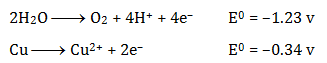So, reaction carried out on anode, which has high SOP value.

Question 21: Which one of the following electronic arrangements is absurd?

1. a. n = 3, l = 1, m = –1
2. b. n = 3, l = 0, m = 0
3. c. n = 2, l = 0, m = –1
4. d. n = 2, l = 1, m = 0

Solution:

Quantum number set n = 2, l = 0, m = –1 is not possible (not valid) since value of m ≤ +l to –l.

Question 22: The quantity hv/kB corresponds to

1. a. Wavelength
2. b. Velocity
3. c. Temperature
4. d. Angular momentum

Solution:

hv/kB = (3/2)T (it represents temperature)

Question 23: In the crystalline solid MSO4.nH2O of molar mass 250 g mol–1, the percentage of anhydrous salt is 64 by weight. The value of n is

1. a. 2
2. b. 3
3. c. 5
4. d. 7

Solution:

Mass of anhydrous MSO4 salt = 250 × (64/100) = 160 gm/mole

The total mass of H2O is MSO4.nH2O

= 250 – 160 = 90 gm/mole

So value of n = (90/18) = 5

Question 24: At S.T.P., the volume of 7.5 g of a gas is 5.6 L. The gas is

1. a. NO
2. b. N2O
3. c. CO
4. d. CO2

Solution:

(A) For finding which gas is it, we have to find molar mass.

Since, At STP weight of 5.6 L gas = 7.5 gm (given)

At STP weight of 22.4 L gas =(7.5/5.6) × 22.4 = 30 gm/mole

And we know, molar mass of NO is 30 gm/mole

Question 25: The half-life period of 53I125 is 60 days. The radioactivity after 180 days will be

1. a. 25 %
2. b. 12.5 %
3. c. 33.3 %
4. d. 3.0 %

Solution:

t 1/2 = 60 days (given)

Radioactivity after t time Nt = N0/(2n) … (1)

& we know, n = t/t 1/2

So,n = (180/60) ; n = 3

Putting value of n in eq. (1)

Nt = N0/(23) = N0/8 = 0.125 N0

So, radioactivity after 180 days = 12.5 %

Question 26: Consider the radioactive disintegration

82A210→ B → C →82D206

The sequence of emission can be

1. a. β, β, β
2. b. α, α, β
3. c. β, β, γ
4. d. β, β, α

Solution: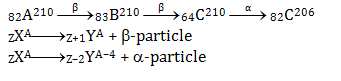Question 27: The second Ionisation energy of the following elements follows the order

1. a. Zn > Cd < Hg
2. b. Zn > Cd > Hg
3. c. Cd > Hg < Zn
4. d. Zn < Cd < Hg

Solution:

2nd I.E. order: Cd < Zn < Hg

So, Zn > Cd < Hg

Element 2nd I.E. (kJ/mole)

Zn 1734

Cd 1631

Hg 1809

Question 28: The melting points of (i) BeCl2 (ii) CaCl2 and (iii) HgCl2 follow the order

1. a. i < ii < iii
2. b. iii < i < ii
3. c. i < iii < ii
4. d. ii < i < iii

Solution:

According to covalent character

Melting points ∝1/ covalent character

Melting points HgCl2 = 276°C

BeCl2 = 399°C

CaCl2 = 775°C

Melting point order = HgCl2< BeCl2< CaCl2

Question 29: Which of these species will have non-zero magnetic moment?

1. a. Na+
2. b. Mg
3. c. F
4. d. Ar+

Solution:

By writing configurations we can find number of unpaired electrons.

If the number of unpaired electrons is 0

Then, magnetic moment will also be 0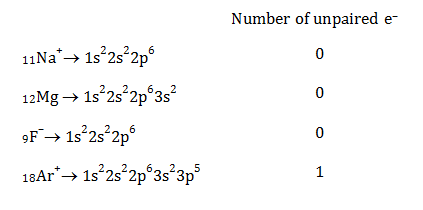Question 30: The first electron affinity of C, N and O will be of the order

1. a.C < N < O
2. b. N < C < O
3. c. C < O < N
4. d. O < N < C

Solution:

1st electron affinity order: N < C < O

According to electronic configuration,

N = 1s2 2s2 2p3

Half filled orbitals are more stable.

E.A. kJ/mole [C = 121.77, N = –6.8, O = 140]

Question 31: Oxidation of allyl alcohol with a peracid gives a compound of molecular formula C3H6O2, which contains an asymmetric carbon atom. The structure of the compound is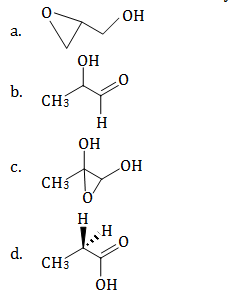Solution: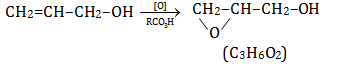An epoxide ring formation will take place.

Question 32: The total number of isomeric liner dipeptide which can be synthesized from racemic alanine is

1. a. 1
2. b. 2
3. c. 3
4. d. 4

Solution: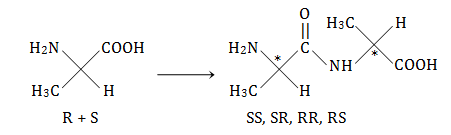Dipeptide has two chiral carbons and both side unsymmetrical. Hence RR, RS, SR, SS is possible.

Question 33: The kinetic study of a reaction like vA→P at 300 K provides the following curve. Where concentration is taken in mol dm–3 and time in min.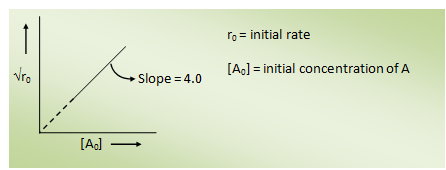1. a. n = 0, k = 4.0mol dm–3 min–1
2. b. n = 1/2, k = 2.0 mol 1/2 dm 3/4dm–3 min–1
3. c. n = 1, k = 8.0 min–1
4. d. n = 2, k = 16.0 dm3 mol–1 min–1

Solution:

Rate = k(A)n

According to graph (n = 2)

Slope =(Rate)1/2/(A) = 4

K =Rate/(A)2 ;Rate/(A)2 = (4)2

= 16

Question 34: At constant pressure, the heat of formation of a compound is not dependent on temperature, when

1. a. ∆Cp = 0
2. b. ∆CV = 0
3. c. ∆Cp> 0
4. d. ∆Cp< 0

Solution:

For reaction: (According to Kirchhoff’s equation)

∆H2 = ∆H1 + ∆Cp(∆T)

When ∆Cp = 0

Then, ∆Hf will not depend on temperature.

Question 35: A copper coin was electroplated with Zn and then heated at a high temperature until there is a change in colour. What will be the resulting colour?

1. a. White
2. b. Black
3. c. Silver
4. d.Golden

Solution:

If these coins are heated, the zinc will diffuse into the copper layer, producing a surface alloy of zinc and copper. These alloys are brasses. Copper also oxidizes when heated in air, producing a black layer of copper-oxide (CuO).

Question 36: The compounds(s), capable of producing achiral compound on heating at 100°C is/are: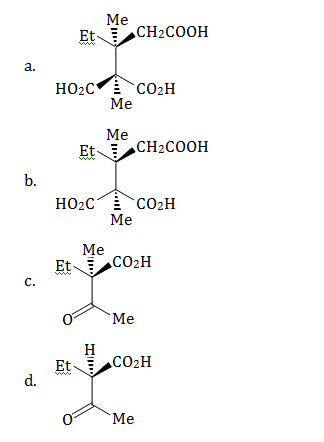Solution:

On heating the compound CO2 gas will evolve out.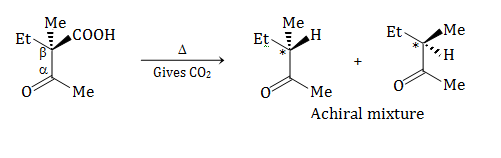In product chiral carbon atom is present hence, R and S structure will be there.

Question 37: Haloform reaction with I2 and KOH will be responded by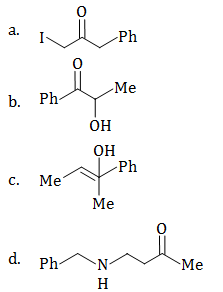Solution:

I2/KOH reacts on methyl ketones or 2° alcohols.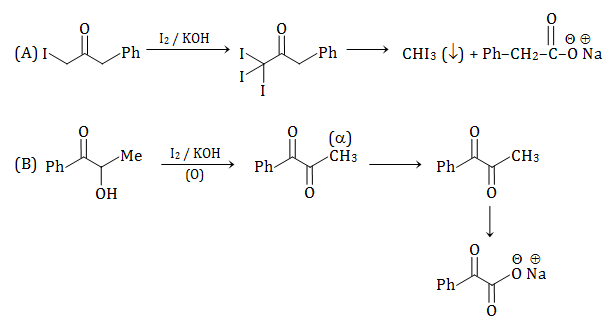2° alcohols can be converted to ketones.

Question 38: Identify the correct statement(s):

1. a. The oxidation number of Cr in CrO5 is +6.
2. b. ΔH > ΔU for the reaction N2O4(g)→2NO2(g). Provided both gases behave ideally.
3. c. pH of 0.1N H2SO4 is less than that of 0.1 N HCl at 25°C
4. d. (RT/F) = 0.0591 volt at 25°C

Solution: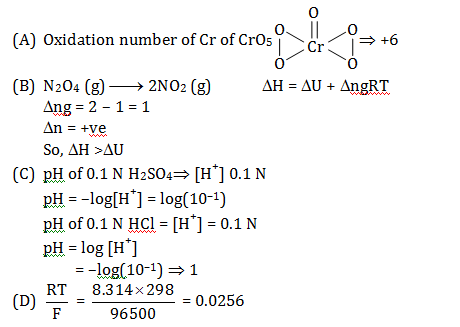Question 39: Compounds with spin-only magnetic moment equivalent to five unpaired electrons are:

1. a. K4[Mn(CN)6]
2. b. [Fe(H2O)6]Cl3
3. c. K3[FeF6]
4. d. K4[MnF6]

Solution:

(A) In K4[Mn(CN)6], Mn has +20.5 and Mn2+ = [Ar] 3d54s0, and CNis a strong field ligand pairing takes place and the complex has only one unpaired electron.

(B) In [Fe(H2O)6]Cl3, Fe has +30.5 and Fe 3+ = [Ar]3d54s0, the number of unpaired electron = 5. (H2O is a weak field ligand thus no pairing)

(C) In K3[FeF6], Fe has +30.5 and Fe3+ = [Ar]3d54s0, thus number of unpaired electron = 5. (F is a weak field ligand thus no pairing).

(D)In K4[MnF6], Mn has 0.5 of +2 thus Mn 2+ = [Ar]3d54s0 is number of unpaired electron = 5.

F is a weak field ligand thus no pairing.

Question 40: Which of the following chemical may be used to identify three unlabelled beakers containing conc. NaOH, conc. H2SO4 and water?

1. a. NH4NO3
2. b. NaCl
3. c. (NH4)2CO3
4. d. HCOONa

Solution:

(A)NH4NO3 will evolve NH3, pungent smelling gas with NaOH, NO2 brown gas with conc. H2SO4 and no reaction in water, thus NH4NO3 can label all three beakers.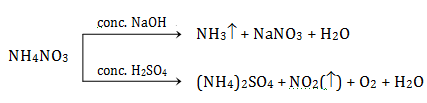(C) (NH4)2CO3 will evolve NH3 pungent-smelling gas with NaOH, effervescence of CO2 with conc. H2SO4 and no reaction with water, thus (NH4)2CO3 can label all three beakers.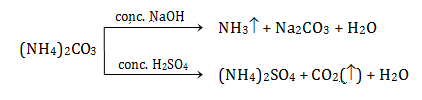### WBJEE 2019 Chemistry Question Paper with Solutions